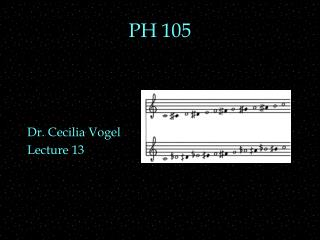DownloadDownload PresentationPH 105

# PH 105

Télécharger la présentation## PH 105

- - - - - - - - - - - - - - - - - - - - - - - - - - - E N D - - - - - - - - - - - - - - - - - - - - - - - - - - -
##### Presentation Transcript

1. PH 105 Dr. Cecilia Vogel Lecture 13

2. OUTLINE • Timbre and graphs: • Time graph • Spectrum graph • Spectrogram • Envelope • scales • units • interval factors

3. Fourier Analysis • Fourier Synthesis meant from the spectrum, build the • Fourier Analysis means • from the waveform, find the • Let’s let the computer do it. • This yields a spectrum graph • or ____ graph • Amplitude vs

4. Pitch and Timbre —Graphs • Dominant influences on pitch and timbre: *f1 might be lowest freq, but might not *Often overtones are nf1.

5. Envelope • Envelope is the time variation of the sound • Often divided into: • attack = initial building up of sound • decay = decrease to sustained level • or to zero, if the sound is not sustained • sustain = sound held fairly steady • release = final decrease of sound to zero A D S R

6. ADSR • Attack, decay, and release • Are usually described by the time they last • Sustain • is usually described by the level (amplitude) • But all four may have their own • frequency, spectrum, amplitude, and duration

7. Spectrograms • time graphs • show time variation (x-axis is time) • spectrum graphs • show frequencies in spectrum (x-axis is frequency) • Spectrograms • show both • x-axis is time • y-axis is frequency • Amplitude is shown by heaviness of graphing

8. Bird Song Spectrograms • Top one (a) • three trills • many freq’s — noise-like • Bottom one (b) • first a low tone with many harmonics • rises and falls slightly • then higher, purer tone • falls slightly • finally lower, more noise-like tone

9. Octave • If two pitches • the frequency of one is • fundamental similarity in • often leads to • Octaves are further divided • into cents, semitones, whole tones

10. Logarithmic Frequency Measures

11. Cents • One cent interval has a ratio of 1.0006 • 1 cent above 440Hz is • Can you tell the difference between 440 Hz and 440.25 Hz? • a jnd is a ratio of 1.005 • about 8-9 cents • 10 cent above 440Hz is • Can you tell the difference between 440 Hz and 442.55 Hz? (10 cents)

12. Cents Calculation • Interval, I, in cents is related to the • Example, an octave has a ratio of

13. Semitone • An octave is often • each semitone is a factor of • multiply 440 Hz (an A) by • you’ll get about 880 Hz • Keys on a piano are separated by • 12 semitones in order is a

14. Musical Staff • Musical notes are • the x-axis is • the y-axis is • Fig 8.9 • Only the notes in spaces are written in. • Notes on lines are letters between. • Short lines indicate where sharp/flat would be , graphically.

15. Major Diatonic Scale • Western music uses a ____________ instead. • A major diatonic scale has • (the 8th would be an • The intervals are not all semitones • some are • whole tone is about a factor of (1.06)2 = 1.12 • The intervals in major diatonic scale are • Start with any key on the keyboard. • You’ve played a major diatonic scale.

16. Example • Key of C (major diatonic scale) • play • CDEFGAB • C to D is a • C#/Db is between • similarly with • E to F is a

17. Scale on Piano • one octave on keyboard • ignore the gray for now

18. Summary • Envelope — ADSR • equal pitch intervals are equal frequency factors • jnd, cents, semitone, whole tone, octaves • Scales • chromatic, 12 notes, 1 semitone apart • major diatonic, 7 notes, whole and semitone intervals • Staff